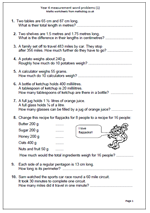# Year 4 measurement word problemsWord problems can cause children quite a few problems as they have to work out what mathematical operation(s) to carry out to reach the right answers. This page is a set of word problems all to do with measurement, including time.

In order to reach the correct answers children need to be able to calculate and know about metric measurements. For example, question 1 asks:

Two tables are 65 cm and 87 cm long. What is their total length in metres.

Not only do they have to add 65 and 87 to get 152, but then convert this to 1.52 metres.

Other questions involve calculating in quarters and doubling the weight of the ingredients for making flapjacks; an early introduction to ratio.

Measuring word problems (1)